## Multi-loop Circuits and Kirchoff's Rules

7-13-99

Before talking about what a multi-loop circuit is, it is helpful to define two terms, junction and branch.

A junction is a point where at least three circuit paths meet.

A branch is a path connecting two junctions.

In the circuit below, there are two junctions, labeled a and b. There are three branches: these are the three paths from a to b.### Multi-loop circuits

In a circuit involving one battery and a number of resistors in series and/or parallel, the resistors can generally be reduced to a single equivalent resistor. With more than one battery, the situation is trickier. If all the batteries are part of one branch they can be combined into a single equivalent battery. Generally, the batteries will be part of different branches, and another method has to be used to analyze the circuit to find the current in each branch. Circuits like this are known as multi-loop circuits.

Finding the current in all branches of a multi-loop circuit (or the emf of a battery or the value of a resistor) is done by following guidelines known as Kirchoff's rules. These guidelines also apply to very simple circuits.

Kirchoff's first rule : the junction rule. The sum of the currents coming in to a junction is equal to the sum leaving the junction. (Basically this is conservation of charge)

Kirchoff's second rule : the loop rule. The sum of all the potential differences around a complete loop is equal to zero. (Conservation of energy)

There are two different methods for analyzing circuits. The standard method in physics, which is the one followed by the textbook, is the branch current method. There is another method, the loop current method, but we won't worry about that one.

### The branch current method

To analyze a circuit using the branch-current method involves three steps:

1. Label the current and the current direction in each branch. Sometimes it's hard to tell which is the correct direction for the current in a particular loop. That does NOT matter. Simply pick a direction. If you guess wrong, youąll get a negative value. The value is correct, and the negative sign means that the current direction is opposite to the way you guessed. You should use the negative sign in your calculations, however.

2. Use Kirchoff's first rule to write down current equations for each junction that gives you a different equation. For a circuit with two inner loops and two junctions, one current equation is enough because both junctions give you the same equation.

3. Use Kirchoff's second rule to write down loop equations for as many loops as it takes to include each branch at least once. To write down a loop equation, you choose a starting point, and then walk around the loop in one direction until you get back to the starting point. As you cross batteries and resistors, write down each voltage change. Add these voltage gains and losses up and set them equal to zero.

When you cross a battery from the - side to the + side, that's a positive change. Going the other way gives you a drop in potential, so that's a negative change.

When you cross a resistor in the same direction as the current, that's also a drop in potential so it's a negative change in potential. Crossing a resistor in the opposite direction as the current gives you a positive change in potential.

### An example

Running through an example should help clarify how Kirchoff's rules are used. Consider the circuit below: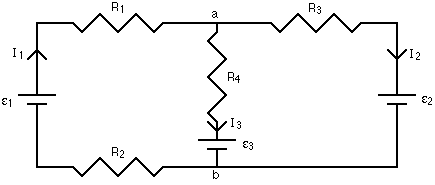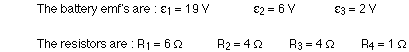Step 1 of the branch current method has already been done. The currents have been labeled in each branch of the circuit, and the directions are shown with arrows. Again, you don't have to be sure of these directions at this point. Simply choose directions, and if any of the currents come out to have negative signs, all it means is that the direction of that current is opposite to the way you've shown on your diagram.

Applying step 2 of the branch current method means looking at the junctions, and writing down a current equation. At junction a, the total current coming in to the junction equals the total current flowing away. This gives:

at junction a : I1 = I2 + I3

If we applied the junction rule at junction b, we'd get the same equation. So, applying the junction rule at one of the junctions is all we need to do. In some cases you will need to get equations from more than one junction, but you'll never need to get an equation for every junction.

There are three unknowns, the three currents, so we need to have three equations. One came from the junction rule; the other two come from going to step 3 and applying the loop rule. There are three loops to use in this circuit: the inside loop on the left, the inside loop on the right, and the loop that goes all the way around the outside. We just need to write down loop equations until each branch has been used at least once, though, so using any two of the three loops in this case is sufficient.

When applying the loop equation, the first step is to choose a starting point on one loop. Then walk around the loop, in either direction, and write down the change in potential when you go through a battery or resistor. When the potential increases, the change is positive; when the potential decreases, the change is negative. When you get back to your starting point, add up all the potential changes and set this sum equal to zero, because the net change should be zero when you get back to where you started.

When you pass through a battery from minus to plus, that's a positive change in potential, equal to the emf of the battery. If you go through from plus to minus, the change in potential is equal to minus the emf of the battery.

Current flows from high to low potential through a resistor. If you pass through a resistor in the same direction as the current, the potential, given by IR, will decrease, so it will have a minus sign. If you go through a resistor opposite to the direction of the current, you're going from lower to higher potential, and the IR change in potential has a plus sign.

Keeping all this in mind, let's write down the loop equation for the inside loop on the left side. Picking a starting point as the bottom left corner, and moving clockwise around the loop gives: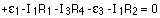Make sure you match the current to the resistor; there is one current for each branch, and a loop has at least two branches in it.

The inner loop on the right side can be used to get the second loop equation. Starting in the bottom right corner and going counter-clockwise gives: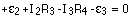Plugging in the values for the resistances and battery emf's gives, for the three equations: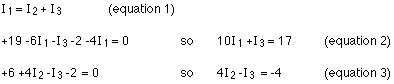The simplest way to solve this is to look at which variable shows up in both loop equations (equations 2 and 3), solve for that variable in equation 1, and substitute it in in equations 2 and 3.

Rearranging equation 1 gives:Substituting this into equation 2 gives: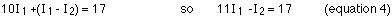Making the same substitution into equation 3 gives: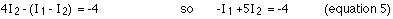This set of two equations in two unknowns can be reduced to one equation in one unknown by multiplying equation 4 by 5 (the number 5, not equation 5!) and adding the result to equation 5.Substituting this into equation 5 gives:

I2 = ( -4 + 1.5 ) / 5 = -0.5 A

The negative sign means that the current is 0.5 A in the direction opposite to that shown on the diagram. Solving for the current in the middle branch from equation 1 gives:

I3 = 1.5 - (-0.5) = 2.0 A

An excellent way to check your answer is to go back and label the voltage at each point in the circuit. If everything is consistent, your answer is fine. To label the voltage, the simplest thing to do is choose one point to be zero volts. It's just the difference in potential between points that matters, so you can define one point to be whatever potential you think is convenient, and use that as your reference point. My habit is to set the negative side of one of the batteries to zero volts, and measure everything else with respect to that.In this example circuit, when the potential at all the points is labeled, everything is consistent. What this means is that when you go from junction b to junction a by any route, and figure out what the potential at a is, you get the same answer for each route. If you got different answers, that would be a big hint that you did something wrong in solving for the currents. Note also that you have to account for any of the currents coming out to be negative, and going the opposite way from what you had originally drawn.

One final note: you can use this method of circuit analysis to solve for more things than just the current. If one or more of the currents was known (maybe the circuit has an ammeter or two, measuring the current magnitude and direction in one or two branches) then an unknown battery emf or an unknown resistance could be found instead.

### Meters

It is often useful to measure the voltage or current in a circuit. A voltmeter is a device used to measure voltage, while a meter measuring current is an ammeter. Meters are either analog or digital devices. Analog meters show the output on a scale with a needle, while digital devices produce a digital readout. Analog voltmeters and ammeters are both based on a device called a galvanometer. Because this is a magnetic device, we'll come back to that in the next chapter. Digital voltmeters and ammeters generally rely on measuring the voltage across a known resistor, and converting that voltage to a digital value for display.

### VoltmetersResistors in parallel have the same voltage across them, so if you want to measure the voltage across a circuit element like a resistor, you place the voltmeter in parallel with the resistor. The voltmeter is shown in the circuit diagram as a V in a circle, and it acts as another resistor. To prevent the voltmeter from changing the current in the circuit (and therefore the voltage across the resistor), the voltmeter must have a resistance much larger than the resistor's. With a large voltmeter resistance, hardly any of the current in the circuit makes a detour through the meter.

### Ammeters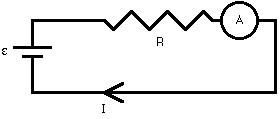Remember that resistors in series have the same current flowing through them. An ammeter, then, must be placed in series with a resistor to measure the current through the resistor. On a circuit diagram, an ammeter is shown as an A in a circle. Again, the ammeter acts as a resistor, so to minimize its impact on the circuit it must have a small resistance relative to the resistance of the resitor whose current is being measured.

### RC Circuits

Resistors are relatively simple circuit elements. When a resistor or a set of resistors is connected to a voltage source, the current is constant. If a capacitor is added to the circuit, the situation changes. In a simple series circuit, with a battery, resistor, and capacitor in series, the current will follow an exponential decay. The time it takes to decay is determined by the resistance (R) and capacitance (C) in the circuit.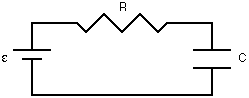A capacitor is a device for storing charge. In some sense, a capacitor acts like a temporary battery. When a capacitor is connected through a resistor to a battery, charge from the battery is stored in the capacitor. This causes a potential difference to build up across the capacitor, which opposes the potential difference of the battery. As this potential difference builds, the current in the circuit decreases.

If the capacitor is connected to a battery with a voltage of Vo, the voltage across the capacitor varies with time according to the equation: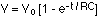The current in the circuit varies with time according to the equation: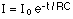Graphs of voltage and current as a function of time while the capacitor charges are shown below.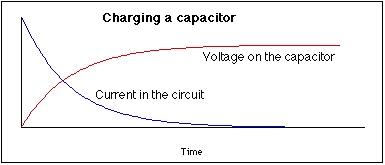The product of the resistance and capacitance, RC, in the circuit is known as the time constant. This is a measure of how fast the capacitor will charge or discharge.

After charging a capacitor with a battery, the battery can be removed and the capacitor can be used to supply current to the circuit. In this case, the current obeys the same equation as above, decaying away exponentially, and the voltage across the capacitor will vary as: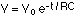Graphs of the voltage and current while the capacitor discharges are shown here. The current is shown negative because it is opposite in direction to the current when the capacitor charges.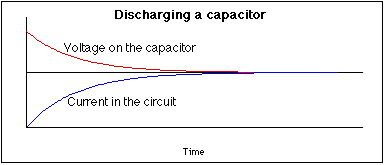### Currents in nerve cells

In the human body, signals are sent back and forth between muscles and the brain, as well as from our sensory receptors (eyes, ears, touch sensors, etc.) to the brain, along nerve cells. These nerve impulses are electrical signals that are transmitted along the body, or axon, of a nerve cell.

The axon is simply a long tube built to carry electrical signals. A potential difference of about 70 mV exists across the cell membrane when the cell is in its resting state; this is due to a small imbalance in the concentration of ions inside and outside the cell. The ions primarily responsible for the propagation of a nerve impulse are potassium (K+) and sodium +.

The potential inside the cell is at -70 mV with respect to the outside. Consider one point on the axon. If the potential inside the axon at that point is raised by a small amount, nothing much happens. If the potential inside is raised to about -55 mV, however, the permeability of the cell membrane changes. This causes sodium ions to enter the cell, raising the potential inside to about +50 mV. At this point the membrane becomes impermeable to sodium again, and potassium ions flow out of the cell, restoring the axon at that point to its rest state.

That brief rise to +50 mV at point A on the axon, however, causes the potential to rise at point B, leading to an ion transfer there, causing the potential there to shoot up to +50 mV, thereby affecting the potential at point C, etc. This is how nerve impulses are transmitted along the nerve cell.